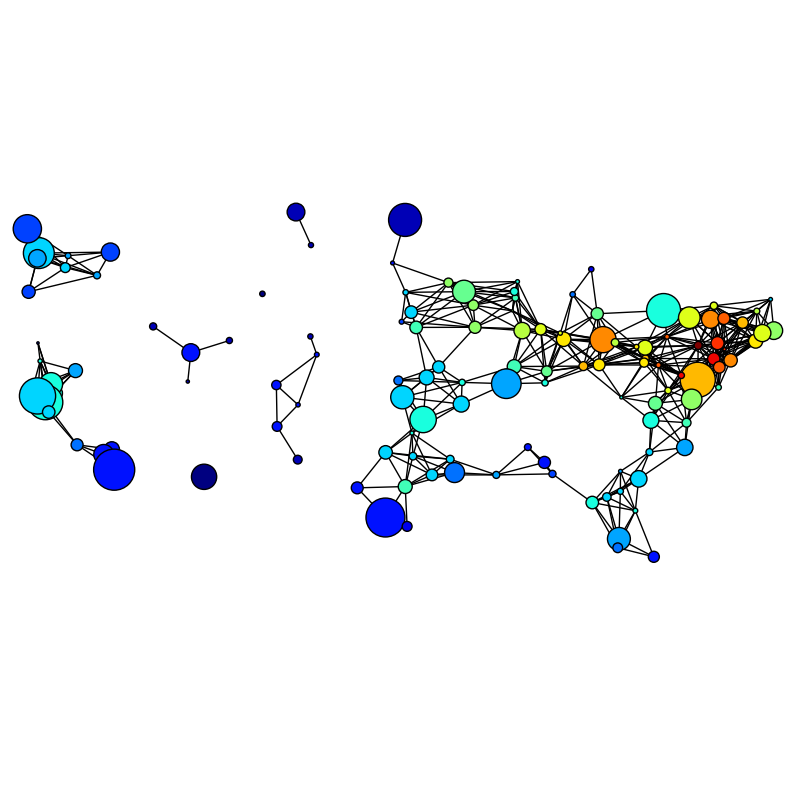Warning

This documents an unmaintained version of NetworkX. Please upgrade to a maintained version and see the current NetworkX documentation.

# Knuth Miles¶#!/usr/bin/env python
"""
An example using networkx.Graph().

miles_graph() returns an undirected graph over the 128 US cities from
the datafile miles_dat.txt. The cities each have location and population
data.  The edges are labeled with the distance betwen the two cities.

This example is described in Section 1.1 in Knuth's book [1,2].

References.
-----------

 Donald E. Knuth,
"The Stanford GraphBase: A Platform for Combinatorial Computing",
ACM Press, New York, 1993.
 http://www-cs-faculty.stanford.edu/~knuth/sgb.html

"""
__author__ = """Aric Hagberg (hagberg@lanl.gov)"""
#    Aric Hagberg <hagberg@lanl.gov>
#    Dan Schult <dschult@colgate.edu>
#    Pieter Swart <swart@lanl.gov>

import networkx as nx

def miles_graph():
""" Return the cites example graph in miles_dat.txt
from the Stanford GraphBase.
"""
# open file miles_dat.txt.gz (or miles_dat.txt)
import gzip
fh = gzip.open('knuth_miles.txt.gz','r')

G=nx.Graph()
G.position={}
G.population={}

cities=[]
line = line.decode()
continue

numfind=re.compile("^\d+")

if numfind.match(line): # this line is distances
dist=line.split()
for d in dist:
i=i+1
else: # this line is a city, position, population
i=1
(city,coordpop)=line.split("[")
cities.insert(0,city)
(coord,pop)=coordpop.split("]")
(y,x)=coord.split(",")

# assign position - flip x axis for matplotlib, shift origin
G.position[city]=(-int(x)+7500,int(y)-3000)
G.population[city]=float(pop)/1000.0
return G

if __name__ == '__main__':
import networkx as nx
import re
import sys

G=miles_graph()

print("digraph has %d nodes with %d edges"\
%(nx.number_of_nodes(G),nx.number_of_edges(G)))

# make new graph of cites, edge if less then 300 miles between them
H=nx.Graph()
for v in G:
for (u,v,d) in G.edges(data=True):
if d['weight'] < 300:

# draw with matplotlib/pylab

try:
import matplotlib.pyplot as plt
plt.figure(figsize=(8,8))
# with nodes colored by degree sized by population
node_color=[float(H.degree(v)) for v in H]
nx.draw(H,G.position,
node_size=[G.population[v] for v in H],
node_color=node_color,
with_labels=False)

# scale the axes equally
plt.xlim(-5000,500)
plt.ylim(-2000,3500)

plt.savefig("knuth_miles.png")
except:
pass